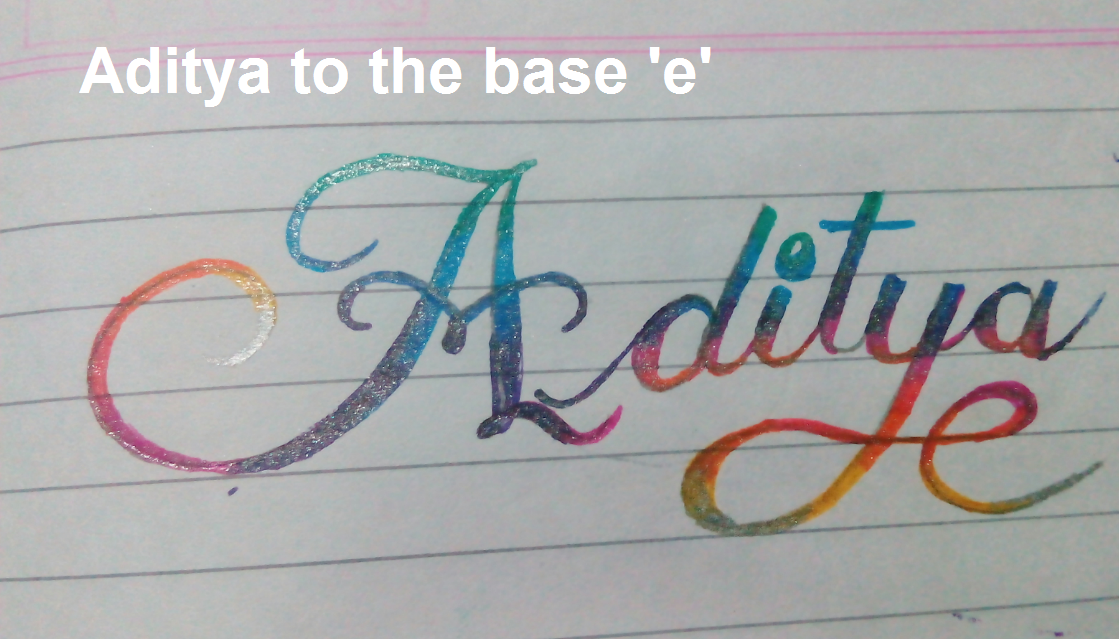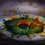# A program of changing the base!Many times when you wish to bash a problem with 'programming-bash', changing base of a number is needed. Of course we can use wolfram, but it'll get irritating if there are too many numbers.

So here, $\color{#3D99F6}{\text{Python comes to our help!}}$

I've written this program in Python 2.7, for getting a decimal number in some other base...

($\text{cb}$ stands for change_base )

  1 2 3 4 5 6 7 8 9 10 11 12 13 14 15 16 17 18 19 from math import log def cb(n,m): """Returns decimal integer n in base m""" if m<1 or m>36 or int(m)!=m: return 0 if m==1: return '1'*n k='' y=int(log(n,m)) while not y<0: p=n/m**y if p<10: k+=str(p) if p>=10: letters=['A','B','C','D','E','F','G','H','I','J','K','L','M','N','O','P','Q','R','S','T','U','V','W','X','Y','Z'] k+=letters[p-10] n=n-p*m**y y-=1 return k 

• This works for bases 1 to 36.

First I had written that using the while loop for $n$, got pretty bad... It left zeroes that come in between, and also I couldn't efficiently assign Alphabets to digits greater than 9.

But now, I've made it shorter and efficient with the while loop for $y$.

Here's the program for the other way, changing a number from other way, into decimal!

  1 2 3 4 5 6 7 8 9 10 11 12 def basedecode(string,m): """Input string in base m, outpt decimal equivalent!""" k=list(string.upper()) p=['A','B','C','D','E','F','G','H','I','J','K','L','M','N','O','P','Q','R','S','T','U','V','W','X','Y','Z'] while any(i in k for i in p) is True: for i in p: if i in k: k[k.index(i)]=str(p.index(i)+10) u=0 for i in xrange(len(k)): u+=int(k[i])*m**(len(k)-i-1) return u 

I want to know if there are any improvements that you can spot, thanks!5 years, 5 months ago

This discussion board is a place to discuss our Daily Challenges and the math and science related to those challenges. Explanations are more than just a solution — they should explain the steps and thinking strategies that you used to obtain the solution. Comments should further the discussion of math and science.

When posting on Brilliant:

• Use the emojis to react to an explanation, whether you're congratulating a job well done , or just really confused .
• Ask specific questions about the challenge or the steps in somebody's explanation. Well-posed questions can add a lot to the discussion, but posting "I don't understand!" doesn't help anyone.
• Try to contribute something new to the discussion, whether it is an extension, generalization or other idea related to the challenge.

MarkdownAppears as
*italics* or _italics_ italics
**bold** or __bold__ bold
- bulleted- list
• bulleted
• list
1. numbered2. list
1. numbered
2. list
Note: you must add a full line of space before and after lists for them to show up correctly
paragraph 1paragraph 2

paragraph 1

paragraph 2

[example link](https://brilliant.org)example link
> This is a quote
This is a quote
    # I indented these lines
# 4 spaces, and now they show
# up as a code block.

print "hello world"
# I indented these lines
# 4 spaces, and now they show
# up as a code block.

print "hello world"
MathAppears as
Remember to wrap math in $$ ... $$ or $ ... $ to ensure proper formatting.
2 \times 3 $2 \times 3$
2^{34} $2^{34}$
a_{i-1} $a_{i-1}$
\frac{2}{3} $\frac{2}{3}$
\sqrt{2} $\sqrt{2}$
\sum_{i=1}^3 $\sum_{i=1}^3$
\sin \theta $\sin \theta$
\boxed{123} $\boxed{123}$

Sort by:

@Aditya Raut Can you please give me some further sources than Codecademy. I guess you also learnt from there at first. But I want to know if there is any "advanced" source like CA. The course at CA is quite limited.

- 5 years, 4 months ago

I didn't learn from codeacademy, I learnt from 1-2 books and mainly, experimenting...

- 5 years, 4 months ago

@Aditya Raut r u a bio student or a cs student. u once posted about a cockroach.

- 5 years, 4 months ago

Well thats now worth a LOL, I am neither bio student nor cs student! officially , I've taken 'Electronics' as a subject for my 12th std board exam...

- 5 years, 4 months ago

Amazing! Y aren't u posting questions these days?

- 5 years, 4 months ago

By the way, I am a 'bio student' in terms of pen and paper but that does not mean I am not a computer science student by heart.

- 5 years, 4 months ago

That is great. Experimenting is the best way to learn. Especially in programming. I learn programming the same way.

- 5 years, 4 months ago

- 5 years, 5 months ago

There is a typo in cb(n,m) line 7. It should be return '1'*n

- 5 years, 4 months ago

The standard way to convert a string in base $b$ to an integer is int(string,b),by the way

- 5 years, 4 months ago

What is just happening!

So all I did was nothing but an $\text{exercise}$ of no real use, just use int(str,b)..... Why does this keep happening with me!

Thank you btw, for telling this amazing shortcut, where'd you learn that?

- 5 years, 4 months ago

I don't exactly remember but I guess stackoverflow.

- 5 years, 4 months ago

An excellent article, I hope that you will read mine that I publish on https://freebooksummary.com/how-does-shakespeare-portray-macbeths-guilt-in-act-2-scene-2-20802 this educational platform where students from all over the world can find a lot of useful information.

- 11 months, 1 week ago

Why don't you check your mail.

- 5 years, 5 months ago

he's in 12th now

- 5 years, 5 months ago